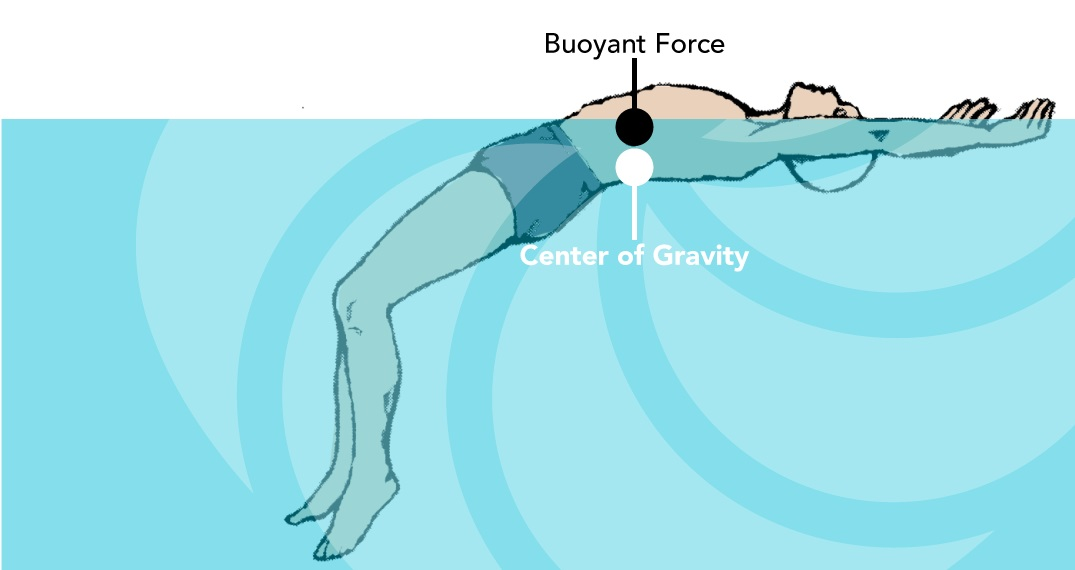#Archimedes’ Principle and Helium Balloons

Buoyancy is the upward force exerted by a fluid that opposes the weight of an object immersed in a particular substance.  Essentially, this is what Archimedes (c.287 BC – c.212 BC) observed when he stated that:

“Any object, wholly or partially immersed in a fluid, is buoyed up by a force equal to the weight of the fluid displaced by the object.”

In a column of fluid, pressure increases with depth as a result of the weight of the overlying fluid.  Thus a column of fluid, or an object submerged within the fluid, experiences an overall greater pressure at the bottom of the column than at the top.

This difference in pressure results in a net force that tends to accelerate an object upwards.  The magnitude of that force is proportional to the difference in the pressure between the top and the bottom of the column, and equivalent to the weight of the fluid that would otherwise occupy the column, i.e. the displaced fluid.

For this reason:

• Any object whose density is greater than that of the fluid in which it is submerged tends to sink.
• If the object is either less dense than the fluid or is shaped appropriately (as in a boat and water), the force can keep the object afloat.

It follows that:

### Buoyancy = Weight of the displaced fluidHow to Stay Afloat in Water

Of course, we can think of a fluid as being either a liquid, or a gas…

# Up and Away

Air is 80% nitrogen.

Since helium weighs 0.1785 grams per litre, and nitrogen weighs 1.2506 grams per litre, 1.25 grams is a good approximation for the weight of a litre of air.

The height to which a balloon rises tends to be stable.

### As a balloon rises, it tends to increase in volume with reducing atmospheric pressure.

But the balloon itself does not expand as much as the air on which it rides.

### The average density of the balloon decreases less than that of the surrounding air.

The weight of the displaced air is reduced.

A rising balloon stops rising when it and the displaced air are equal in weight.  Similarly, a sinking balloon tends to stop sinking.

## A Worked Example about Buoyancy

Here is how you can figure out the lifting capacity of the helium in a spherical helium balloon:

1. Determine the volume of the balloon.

The volume of a sphere is$\frac{4}{3} \times \pi \times r^3$,

where r is the radius of the balloon.

So, we first determine the radius of the sphere.  The radius is half the diameter.

We cube the radius (multiply it by itself twice:$r \times r \times r)$.

Multiply by 4/3 and then multiply by π.

If you are measuring your balloon in feet, that gives you the volume of the balloon in cubic feet.

2. One cubic foot of helium will lift about 28.2 grams.

So, we multiply the volume of the balloon by 28.2.

3. Then, we divide by 448 – the number of grams in a pound – in order to determine the number of pounds that the balloon can lift.

For example, a 20-foot balloon has a radius of 10 feet.

10 x 10 x 10 x 3.14 x 4/3 = 4,186 cubic feet of volume.

4,186 cubic feet x 28.2 grams/cubic feet = 118,064 grams.$\frac{118,064 grams}{448 grams per pound} = 263$ pounds of lifting force.

A 20-foot helium balloon can lift 263 pounds.